J.D. van der Toorn (1997,1998)
A survival guide to survival rates
Updated October 1999

### Introduction

The survival of marine mammals in captivity is often the subject of heated discussions. Interestingly, these discussions usually focus on cetaceans. The discussions are often complicated by a general lack of understanding of the subject matter. This can result in incorrect representation of the available data and comparisons of unrelated parameters. The terminology involved is not straightforward and can be confusing (Fad, 1996).

In this paper, I will discuss the terminology involved, the calculations that must be done to derive survival rates and life expectancies and I will look at the presentation of the data and how that can influence the message. A few examples will be given.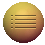### Some definitions

First, let's have a look at some of the terms. All these terms are derived from population dynamics modelling.

• Daily Survival Rate (DSR): the estimated proportion of animals alive on day d that is still alive on day d+1 (DeMaster and Drevenak, 1988). The DSR can also be treated as a probability: then it is defined as the probability that an animal alive on day d will still be alive on day d+1.
• Daily Mortality Rate (DMR): This is the reverse of the DSR: it is the estimated proportion of animals alive on day d that will have died before day d+1.
• Annual Survival Rate (ASR): the estimated proportion of animals alive in year t that is still alive in year t+1 (Olesiuk, et al., 1990). The ASR can, like the DSR, also be treated as a probability: then it is defined as the probability that an animal alive in year t will still be alive in year t+1.
• Annual Mortality Rate (AMR): This is the reverse of the ASR: it is the estimated proportion of animals alive in year t that will have died before year t+1.
• Life expectancy: this is the average age a member of a certain population can be expected to reach. The life expectancy is a function of the ASR.
• Longevity: the maximum age a member of a certain population can reach. This is also sometimes called the maximum life expectancy.
• Average age: this is the average of all the ages of members of a population at a certain point in time (a "snapshot" of the population).
• Animal days:
• For individual animals: the number of days between the time the animal first appeared in the population and either its death or the last day of the sample, if the animal is still alive.
• For populations: the sum of the animal days for all the members of that population.
• Animal years: the same as animal days, but expressed in years instead of days.

Note that all these parameters are calculated for non-calf or non-pup animals (age > 1 year) (Small and DeMaster, 1995). The survival of calves and pups is incorporated in the calculation of reproductive rates. The focus of this paper is on survival rates. The reproductive parameters will be discussed only briefly.

#### Some background on reproductive rates

The following terms can be encountered in discussions about reproduction, as mentioned in a) Olesiuk et al (1990) and b) Wells and Scott (1990):
• Fecundity rate (FEC): This is the number of viable calves born per year as a percentage of the number of mature females or the proportion of mature females, giving birth to viable calves. a,b
• Fertility rate (FER): This is the total number of calves born per year as a percentage of the number of mature females or the proportion of mature females, giving birth a
• Recruitment rate (REC): This is the number of viable calves born per year as a percentage of the total number of animals in the population. b
• Birth rate (BR): This is the number of calves born per year as a percentage of the total number of animals in the population. b
• Neonate mortality (MRn): This is the percentage of calves that die prematurely, i.e. before the age of 0.5 years a or before 1 year of age a. In the following I will use the one-year interval.### Mathematical background

In the following calculations, it is assumed that the ASR and DSR are independent of the actual age of the animals. This is probably not completely accurate. It is likely, that the survival rates differ for different age classes (Barlow and Boveng, 1991). However, the data available to date is insufficient to reliably determine age class dependent survival rates for most marine mammal species (due to a limited sample size) (Small and DeMaster, 1995).

The DSR can be calculated as follows (DeMaster and Drevenak, 1988):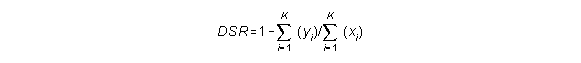where:

• K is the total number of animals in the sample
• yi is 1 if animal i died during the reporting period and 0 if animal i is still alive at the end of the reporting period.
• xi is the number animal days for animal i.

Or, in a simpler form (Small and DeMaster, 1995):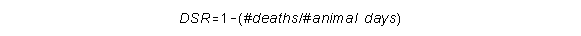The annual survival rate ASR can be derived from the Daily Survival Rate DSR by raising the DSR to the power 365.25 (the average number of days in a year), as follows:The annual mortality rate AMR is, by definition: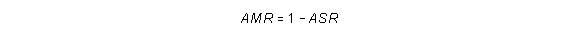If we want to estimate the life expectancy for the population (the average number of years survived), we first need to calculate the total animal years. If we start out with N0 animals, we need to know how many of them are left after one year, two years, etc. After one year, we have: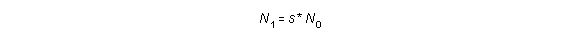In this and the following equations s is the ASR. After two years: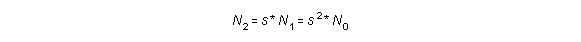And after n years: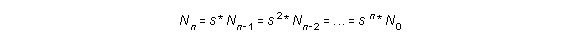If we add them all together, we get: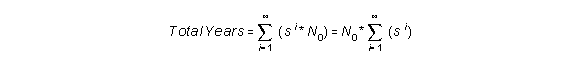If we take infinitely small steps, we can rewrite this formula to:This results in: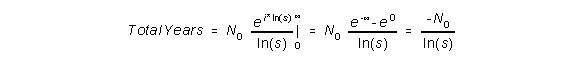To get the life expectancy, which is the average number of years, we need to divide the total number of years by the number of animals in the sample:If we play around a bit with this formula, we can see that the life expectancy is very sensitive to errors. If there is only a small error in the estimate of the ASR, this can result in an error of several years in the derived estimate for the life expectancy. For that reason, life expectancy should not be used when comparing populations, captive or wild (DeMaster and Drevenak, 1988). See the table below for some survival rates, the corresponding life expectancies and the increase in both compared to the previous value in the table. You'll see that a 1% increase in survival rate results in a huge increase in life expectancy estimate for higher ASR values. If you want to play around with some numbers yourself, go to the ASR to Life Expectancy Converter on the Survival Rates Toolkit page.

 ASR ASR increase Life expectancy LE increase 0.920 11.99 0.930 1.09 % 13.78 7.26 % 0.940 1.08 % 16.16 17.27 % 0.950 1.06 % 19.50 20.67 % 0.960 1.05 % 24.50 25.64 % 0.970 1.04 % 32.83 34.00 % 0.980 1.03 % 49.50 50.78 %

#### The reproductive rates

The neonate survival is the complement of neonate mortality:

SRn = 1 - MRn

From this we can calculate the relation between fecundity rate and fertility rate and between birth rate and recruitment rate:

FEC = FER * SRn = FER * ( 1 - MRn )

REC = BR * SRn = BR * ( 1 - MRn )

Where:

• SRn is the neonate survival rate, the percentage of pups or calves surviving to the age of 1 year
• MRn is the neonate mortality rate
• FEC is the fecundity rate
• FER is the fertility rate
• REC is the recruitment rate
• BR is the birth rate

So far, we have not yet mentioned the longevity or maximum age. The reason for this is that there is no direct correlation between survival rate and longevity. Longevity is basically an incidental finding: it is determined by the oldest animal you find in your sample. You can only determine the longevity of a group of animals after all the members of that group have died (Duffield and Wells, 1991).

Sometimes, average age is used in comparing wild and captive populations (Duffield and Wells, 1991). The average age is calculated by adding the ages of all the animals in the population and dividing this by the number of animals. This is however of a very limited use. Such a comparison will only yield useful data if both populations have been stable for a long period of time (no increase or decline) (Barlow and Boveng, 1991). Only in a stable population, the calculation of the average age will yield the same result as the calculation of the life expectancy. If the size of a population is changing however, the average age can shift either way.

Let's consider a declining population. There can be a number of reasons for the decline, such as:

• increase in mortality of older animals. In that case the number of (adult) animals dying will be higher than the number of calves being born into the population. As a result, the younger age classes will be over-represented and the average age will go down.
• decline in birth rate. In that case, an under-representation of the younger age classes can be expected and the average will increase, as has been noted in the Alaskan Northern sea lion population (York, 1994).

Also increases in population size can shift the average age either way:

• if the increase is caused by an increase in birth rate, the population will have an increasing number of young animals and consequently, the average age will go down.
• if the increase is caused by a reduction in mortality, more older animals will remain in the population and the average age will go up.

So, a higher average age does not necessarily mean that a certain population is doing better than another population with a lower average age.